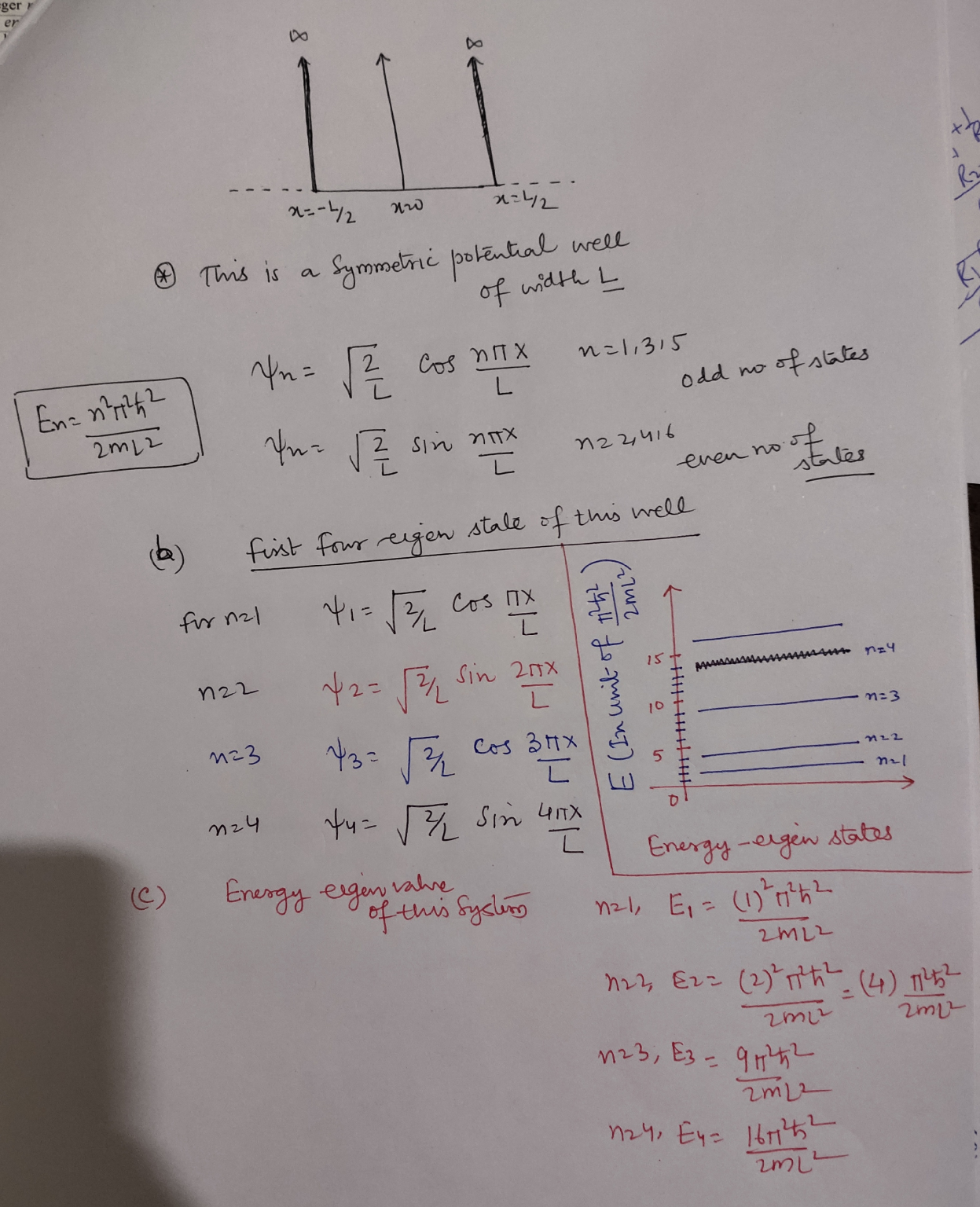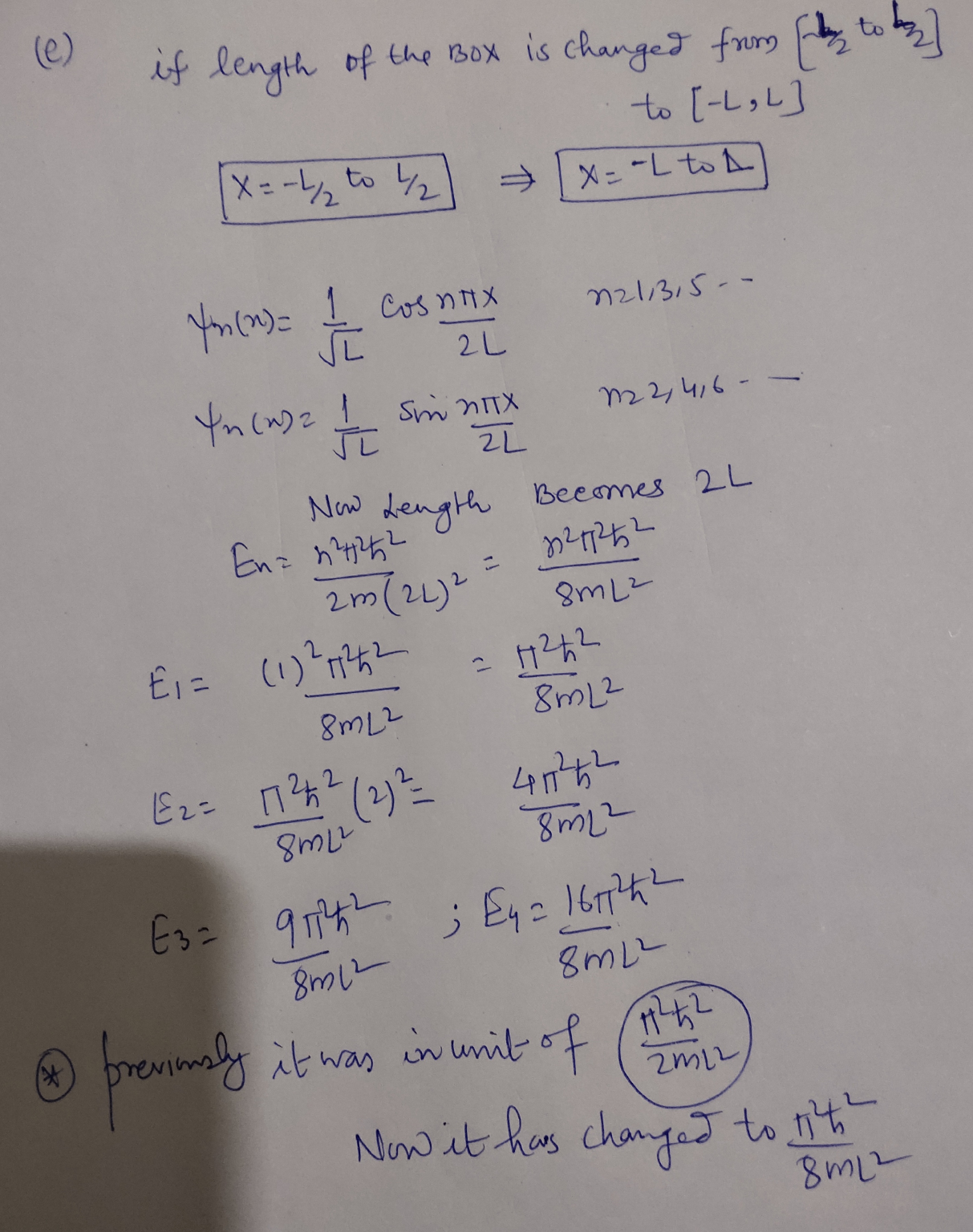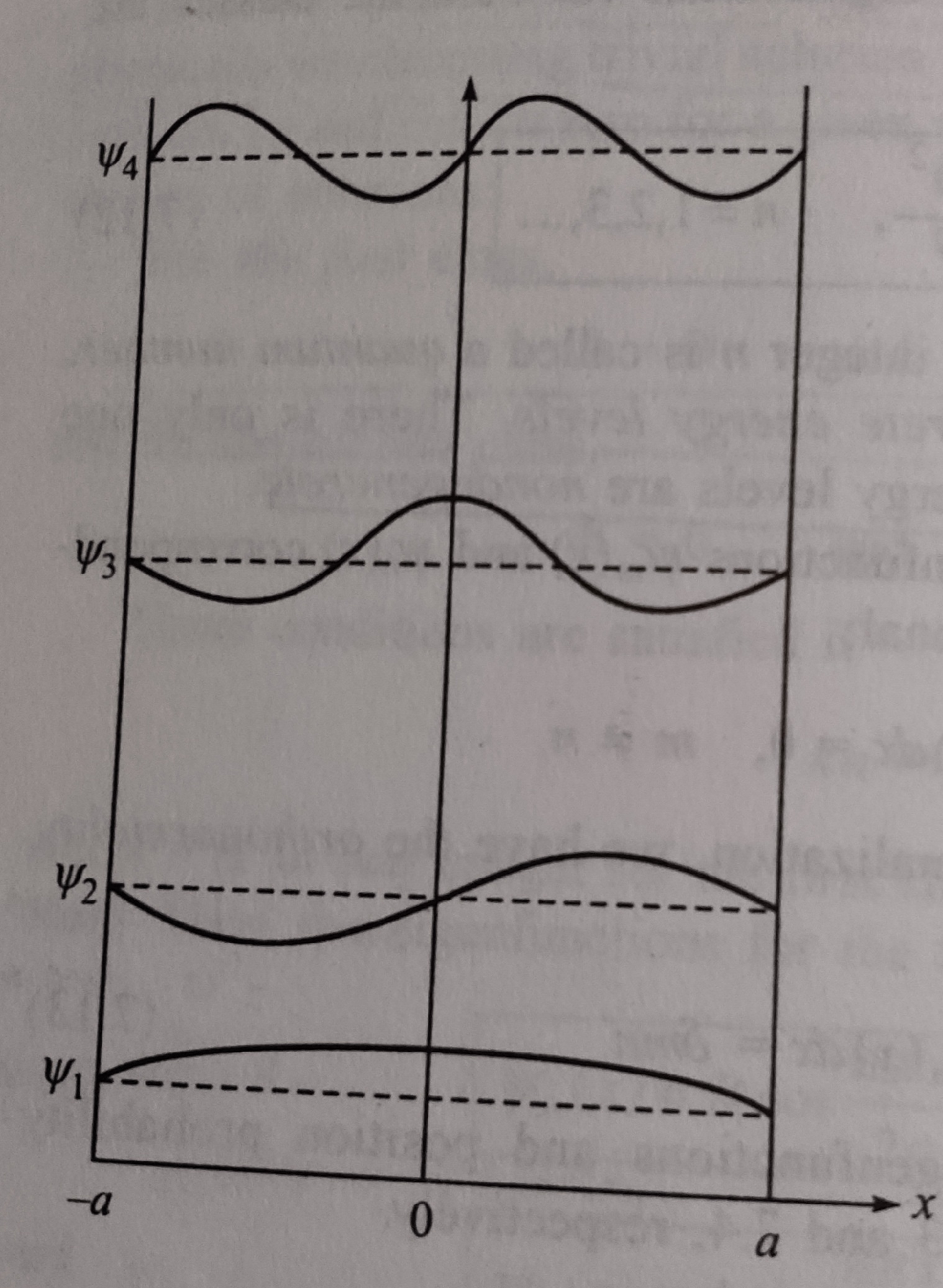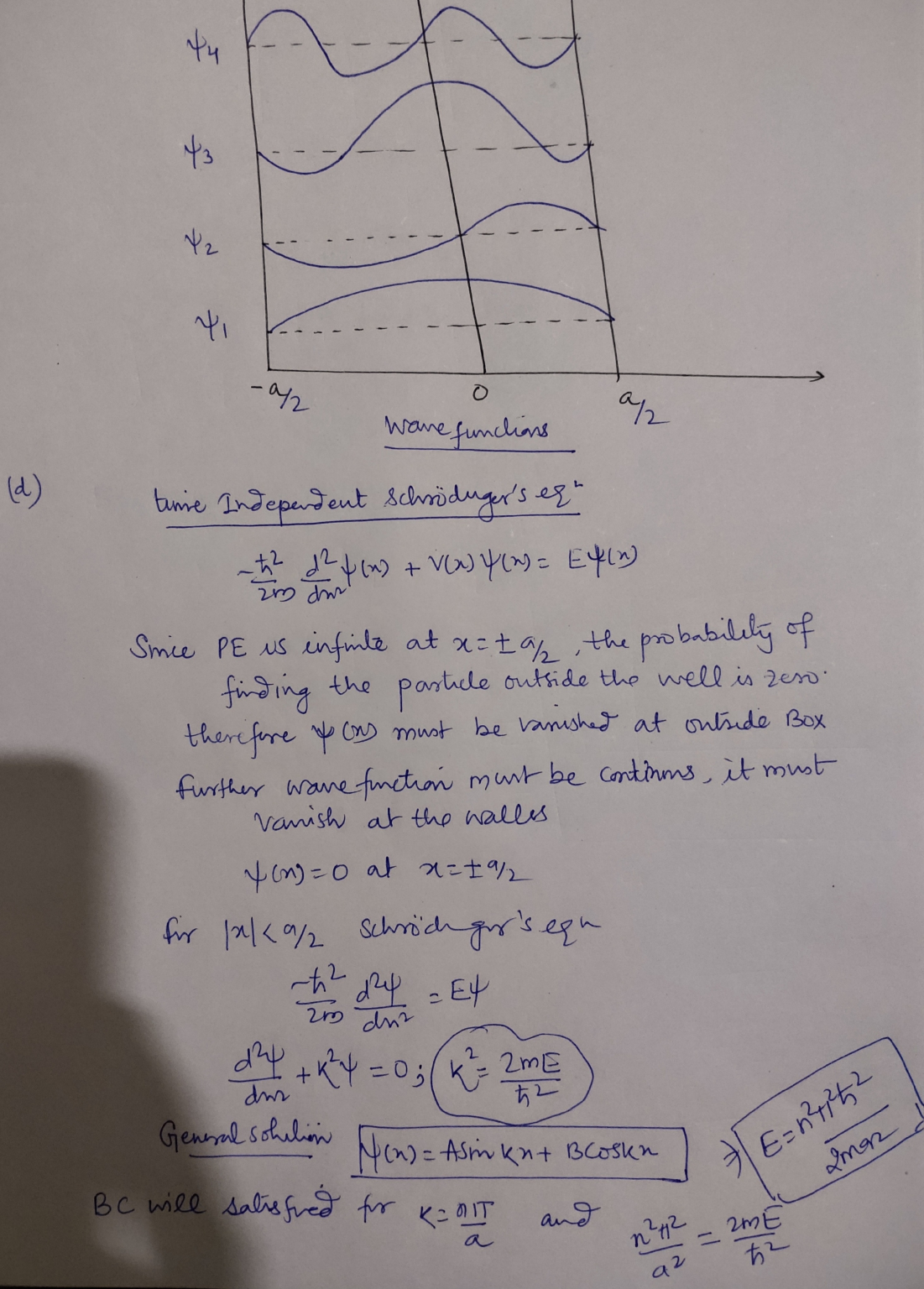In: Physics

# Consider a particle in an infinite square well, but instead of having the well from 0...

Consider a particle in an infinite square well, but instead of having the well from 0 to L as we have done in the past, it is now centered at 0 and the walls are at x = −L/2 and x = L/2.

(a) Draw the first four energy eigenstates of this well.

(b) Write the eigenfunctions for each of these eigenstates.

(c) What are the energy eigenvalues for this system?

(d) Can you find a general expression for the energy eigenstates for this well? (Hint: you should have a different form for even and odd solutions) (e) If instead, the well were from x = −L to x = L, how would these expressions change? Would the energy eigenvalues change? (And if so, how?)

## Solutions

##### Expert Solution#Hi, I am providing you the very detailed solution for all the parts of the question you have asked. My drawing is little weird, I apologize. The picture is taken from my book of Quantum physics. ...which is in public....if you are happy and find this useful please thumbs up. In case, if you have any query regarding the solution please let me know in the comments section below. Thanks!!

## Related Solutions

##### Consider a particle of mass ? in an infinite square well of width ?. Its wave...
Consider a particle of mass ? in an infinite square well of width ?. Its wave function at time t = 0 is a superposition of the third and fourth energy eigenstates as follows: ? (?, 0) = ? 3i?­3(?)+ ?­4(?) (Find A by normalizing ?(?, 0).) (Find ?(?, ?).) Find energy expectation value, <E> at time ? = 0. You should not need to evaluate any integrals. Is <E> time dependent? Use qualitative reasoning to justify. If you measure...
##### A particle in an infinite one-dimensional square well is in the ground state with an energy...
A particle in an infinite one-dimensional square well is in the ground state with an energy of 2.23 eV. a) If the particle is an electron, what is the size of the box? b) How much energy must be added to the particle to reach the 3rd excited state (n = 4)? c) If the particle is a proton, what is the size of the box? d) For a proton, how does your answer b) change?
##### A particle in the infinite square well (width a) starts out being equally likely to be...
A particle in the infinite square well (width a) starts out being equally likely to be found in the first and last third of the well and zero in the middle third. What is the initial (t=0) wave function? Find A and graph the initial wave function. What is the expectation value of x? Show your calculation for the expectation value of x, but then say why you could have just written down the answer. Will you ever find the...
##### Find the energy spectrum of a particle in the infinite square well, with potential U(x) →...
Find the energy spectrum of a particle in the infinite square well, with potential U(x) → ∞ for |x| > L and U(x) = αδ(x) for |x| < L. Demonstrate that in the limit α ≫ hbar^2/mL, the low energy part of the spectrum consists of a set of closely-positioned pairs of energy levels for α > 0. What is the structure of energy spectrum for α < 0?
##### An infinite potential well in one dimension for 0 ≤ x ≤ a contains a particle...
An infinite potential well in one dimension for 0 ≤ x ≤ a contains a particle with the wave function ψ = Cx(a − x), where C is the normalization constant. What is the probability wn for the particle to be in the nth eigenstate of the innite potential well? Find approximate numerical values for w1, w2 and w3.
##### Consider a semi-infinite square well: U(x)=0 for 0 ≤ x ≤ L, U(x)=U0 for x >...
Consider a semi-infinite square well: U(x)=0 for 0 ≤ x ≤ L, U(x)=U0 for x > L, and U(x) is infinity otherwise. Determine the wavefunction for E < Uo , as far as possible, and obtain the transcendental equation for the allowable energies E. Find the necessary condition(s) on E for the solution to exist.
##### Consider a system of N particles in an infinite square well fro, x=0 to x=N*a. find...
Consider a system of N particles in an infinite square well fro, x=0 to x=N*a. find the ground state wave function and ground state energy for A. fermions. B. bosons.
##### For the infinite square-well potential, find the probability that a particle in its second excited state...
For the infinite square-well potential, find the probability that a particle in its second excited state is in each third of the one-dimensional box: 0?x?L/3 L/3?x?2L/3 2L/3?x?L There's already an answer on the site saying that the wavefunction is equal to ?(2/L)sin(2?x/L). My professor gave us this equation, but also gave us the equation as wavefunction = Asin(kx)+Bcos(kx), for specific use when solving an infinite potential well. How do I know which equation to use and when? Thanks
##### For the infinite square-well potential, find the probability that a particle in its fifth excited state...
For the infinite square-well potential, find the probability that a particle in its fifth excited state is in each third of the one-dimensional box: ----------------(0 ≤ x ≤ L/3) ----------------(L/3 ≤ x ≤ 2L/3) ------------------(2L/3 ≤ x ≤ L)
##### Consider two non-interacting particles in an infinite square well. One is in a state ψm, the...
Consider two non-interacting particles in an infinite square well. One is in a state ψm, the other in a state ψn with n≠m. Let’s assume that ψm and ψn are the ground state and 1st excited state respectively and that the two particles are identical fermions. The well is of width 1Å. What is the probability of finding a particle in the 1st excited state in a region of width 0.01Å? Does this change if the particles are distinguishable?